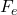## The Millikan oil drop experiment of 1909 allowed Robert A. Millikan to determine the charge of an electron. In the experiment, an oil drop i

Question

The Millikan oil drop experiment of 1909 allowed Robert A. Millikan to determine the charge of an electron. In the experiment, an oil drop is suspended between two charged plated by an electric force that equals the gravitational force acting on the 1.1 x 10-14-kg drop. a) What is the charge on the drop if it remains stationary in an electric field of 1.72 x 105 N/C? b) How many extra electrons are there on this particular oil drop?

in progress 0
2 months 2021-08-12T13:44:48+00:00 1 Answers 0 views 0

a)   q = 6.27 10⁻¹⁹ C
, b)  4 electron

Explanation:

a) In this configuration of the Mullikan experiment, the sum of the forces is zero, let’s use the equilibrium law of / newton– W = 0

q E = mg

q = mg / E

Let’s calculate

q = 1.1 10⁻¹⁴ 9.8 / 1.72 10⁵

q = 6.27 10⁻¹⁹ C

b) Millikan found that the minimum charge that can exist is

q₀ = 1.6 10⁻¹⁹ C

So all charge are an integer multiple of this minimum value.

q = n q₀

n = q / q₀

n = 6.27 10⁻¹⁹ / 1.6 10⁻¹⁹

n = 3.92 = 4

In our drop we have 4 electrons